# Oops...

This website is made for modern browsers. You are seeing this, because your browser is missing a feature or two. Please install the latest update or switch to a modern browser. See you soon.

# Create a sliceform

This tutorial builds upon the result from Generate slices from a 3d model in which we created a sliced solid and arranged those slices in one flat layer. To generate a sliceform, we have to create slots for the opposed slices to interlock. It’s assumed that the slices meet at right angles. The result could be fabricated with a CNC machine

.

## Grasshopper

#### 1

##### Define initial geometric object

To start, we open the result from the tutorial Generate slices from a 3d model and look for the section curves, which are located at the end of step 3 in the Geometry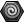Geometry (Geo)
Params  >  Geometry  >  Geometry
Contains a collection of generic geometry
container. Let’s copy and paste this container and start from here.

Our fabricated model should stand without wobbling and therefore we will trim everything underneath a ground plane. This creates a proper bearing area. We create the ground surface with the component Plane Surface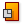Plane Surface (PlaneSrf)
Surface  >  Primitive  >  Plane Surface
Create a plane surface
Inputs
Plane (P)Surface base plane
X Size (X)Dimensions in X direction
Y Size (Y)Dimensions in Y direction
Outputs
Plane (P)Resulting plane surface
and use a domain -100 To 100 as dimensions for each direction.
These dimensions just need to be bigger than the dimensions of our model.
These dimensions just need to be bigger than the dimensions of our model.

#### 2

##### Trim slices with ground surface

The component Split Brep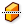Split Brep (Split)
Intersect  >  Shape  >  Split Brep
Split one brep with another.
Inputs
Brep (B)Brep to split
Cutter (C)Cutting shape
Outputs
Result (R)Brep fragments
can be used to cut the section curves at ground surface (the curves at input B will automatically be converted to Breps). At output R we find the resulting pieces, which are positioned either above or below the ground surface. We need the curves above ground. To find them, we need a criterion that separates the surfaces. Here, we check whether the center point of each surface is above the ground plane.

To compute the center points, we use Area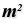Area (Area)
Surface  >  Analysis  >  Area
Solve area properties for breps, meshes and planar closed curves.
Inputs
Geometry (G)Brep, mesh or planar closed curve for area computation
Outputs
Area (A)Area of geometry
Centroid (C)Area centroid of geometry
and find them at output C. Next, we use Deconstruct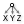Deconstruct (pDecon)
Vector  >  Point  >  Deconstruct
Deconstruct a point into its component parts.
Inputs
Point (P)Input point
Outputs
X component (X)Point {x} component
Y component (Y)Point {y} component
Z component (Z)Point {z} component
to extract the z-coordinates of each point and check if they are Larger Than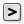Larger Than (Larger)
Maths  >  Operators  >  Larger Than
Larger than (or equal to)
Inputs
First Number (A)Number to test
Second Number (B)Number to test against
Outputs
Larger than (>)True if A > B
… or Equal to (>=)True if A >= B
0 (assuming that the ground plane is has no altitude). Dispatch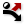Dispatch (Dispatch)
Sets  >  List  >  Dispatch
Dispatch the items in a list into two target lists.
Inputs
List (L)List to filter
Dispatch pattern (P)Dispatch pattern
Outputs
List A (A)Dispatch target for True values
List B (B)Dispatch target for False values
will do the filtering and we find all True cases at output A.

#### 3

##### Find position of slots in the section surfaces

To create a sliceform, we must calculate where the slices meet. For this, we need two lists; each direction of slices in one. After step 2, each section surface has its own branch and we use Trim TreeTrim Tree (Trim)
Sets  >  Tree  >  Trim Tree
Reduce the complexity of a tree by merging the outermost branches.
Inputs
Tree (T)Data tree to flatten
Depth (D)Number of outermost branches to merge
Outputs
Tree (T)Trimmed data tree
to remove the last level. Then we can use Explode Tree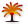Explode Tree (BANG!)
Sets  >  Tree  >  Explode Tree
Extract all the branches from a tree
Inputs
Data (D)Data to explode
Outputs
Branch 0 (-)All data inside the branch at index: 0
Branch 1 (-)All data inside the branch at index: 1
to separate both directions.

To solve the intersection for the slices, we use Brep | Brep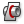Brep | Brep (BBX)
Intersect  >  Physical  >  Brep | Brep
Solve intersection events for two Breps.
Inputs
Brep A (A)First Brep
Brep B (B)Second Brep
Outputs
Curves (C)Intersection curves
Points (P)Intersection points
and we graft one of the inputs to ensure that all surfaces are compared. Of course, not all slices meet and we have to use Clean Tree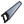Clean Tree (Clean)
Sets  >  Tree  >  Clean Tree
Removed all null and invalid items from a data tree.
Inputs
Remove Nulls (N)Remove null items from the tree.
Remove Invalid (X)Remove invalid items from the tree.
Remove Empty (E)Remove empty branches from the tree.
Tree (T)Data tree to clean
Outputs
Tree (T)Spotless data tree
to remove empty branches that had no intersection event. The component does not do this by default and we need to manually assign True to input E.

To to find a point on the line that was generated by the intersection, we use Point On Curve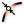Point On Curve (CurvePoint)
Curve  >  Analysis  >  Point On Curve
Evaluates a curve at a specific location
. This defines the depth of the slots and can be the midpoint, but it does not have to.

#### 4

##### Create trimming objects for the slots

After finding the position of the slots, we need to set their dimensions. For this, we use a Domain Box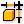Domain Box (Box)
Surface  >  Primitive  >  Domain Box
Create a box defined by a base plane and size domains.
Inputs
Base (B)Base plane
X (X)Domain of the box in the {x} direction.
Y (Y)Domain of the box in the {y} direction.
Z (Z)Domain of the box in the {z} direction.
Outputs
Box (B)Resulting box
. The center of the box will be on the intersection line between the slices and therefore the box needs to expand half of the material thickness into each direction. In this example, we assume that everything was drawn in centimeter and that the material thickness will be 2 mm. This means the boxes will expand 0.1 in each direction. To create a domain from -0.1 to 0.1, we also need to get the Negative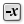Negative (Neg)
Maths  >  Operators  >  Negative
Compute the negative of a value.
Inputs
Value (x)Input value
Outputs
Result (y)Output value
value. Both values are connected to a Construct Domain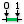Construct Domain (Dom)
Maths  >  Domain  >  Construct Domain
Create a numeric domain from two numeric extremes.
Inputs
Domain start (A)Start value of numeric domain
Domain end (B)End value of numeric domain
Outputs
Domain (I)Numeric domain between {A} and {B}
component, which will create the domain that we use for the X and Y input of our Domain BoxDomain Box (Box)
Surface  >  Primitive  >  Domain Box
Create a box defined by a base plane and size domains.
Inputs
Base (B)Base plane
X (X)Domain of the box in the {x} direction.
Y (Y)Domain of the box in the {y} direction.
Z (Z)Domain of the box in the {z} direction.
Outputs
Box (B)Resulting box
.

When setting the height of the Domain Box, we have to make sure that it reaches from the contact point of the opposed slots to the edge of the material. Also, we need one box oriented in positive z-direction and one in negative. For this, we use Consecutive Domains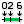Consecutive Domains (Consec)
Maths  >  Domain  >  Consecutive Domains
Create consecutive domains from a list of numbers
Inputs
Numbers (N)Numbers for consecutive domains
Outputs
Domains (D)Domains describing the spaces between the numbers
and assign False to input A, which will deactivate the values being added to a sum-total. To define the heights, we use a PanelPanel
Params  >  Input  >  Panel
A panel for custom notes and text values
and active Multiline Data. We enter the values -10, 0 and 10 and connect it to input A. The resulting Domains are then connected to the Domain Box as input Z.

#### 5

##### Cut slots for interlocking

When cutting the slots for the slices to interlock, we need one slot to face upward and the other to face downward. To select the boxes in once direction, we append a List Item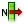List Item (Item)
Sets  >  List  >  List Item
Retrieve a specific item from a list.
Inputs
List (L)Base list
Index (i)Item index
Wrap (W)Wrap index to list bounds
Outputs
Item (i)Item at {i'}
to Domain BoxDomain Box (Box)
Surface  >  Primitive  >  Domain Box
Create a box defined by a base plane and size domains.
Inputs
Base (B)Base plane
X (X)Domain of the box in the {x} direction.
Y (Y)Domain of the box in the {y} direction.
Z (Z)Domain of the box in the {z} direction.
Outputs
Box (B)Resulting box
. Then, we add another input +1 via ZUI to select the second direction.

To cut the slots into the slices, we use Trim Solid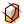Trim Solid (Trim)
Intersect  >  Shape  >  Trim Solid
Cut holes into a shape with a set of solid cutters.
Inputs
Shape (S)Shape to trim
Cutters (T)Trimming shapes
Outputs
Result (R)Shape with holes
. Here, we attach one of the branches that holds the section surfaces (from Explode Tree in step 3) to input S and graft this input. At Input T we attach one set of boxes and flatten it. We repeat the same with another Trim SolidTrim Solid (Trim)
Intersect  >  Shape  >  Trim Solid
Cut holes into a shape with a set of solid cutters.
Inputs
Shape (S)Shape to trim
Cutters (T)Trimming shapes
Outputs
Result (R)Shape with holes
component for the other direction.

#### 6

##### Create a seamless edge for each slice

After creating the slots in the slices, we will inject them into the algorithm from Generate slices from a 3d model to arrange the slices on a single plane for fabrication. Unlike in that tutorial, we now have independent lists and surfaces instead of outline curves. To fix the first, we use Entwine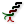Entwine (Entwine)
Sets  >  Tree  >  Entwine
Flatten and combine a collection of data streams
Inputs
Branch {0;0} ({0;0})Data to entwine
Branch {0;1} ({0;1})Data to entwine
Branch {0;2} ({0;2})Data to entwine
Outputs
Result (R)Entwined result
and connect the slices from both directions. After zooming in, we can remove the redundant input.

To convert the surfaces into curves, we attach a Brep Edges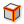Brep Edges (Edges)
Surface  >  Analysis  >  Brep Edges
Extract the edge curves of a brep.
Inputs
Brep (B)Base Brep
Outputs
Naked (En)Naked edge curves
Interior (Ei)Interior edge curves
Non-Manifold (Em)Non-Manifold edge curves
component and use Join Curves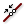Join Curves (Join)
Curve  >  Util  >  Join Curves
Join as many curves as possible
Inputs
Curves (C)Curves to join
Preserve (P)Preserve direction of input curves
Outputs
Curves (C)Joined curves and individual curves that could not be joined.
to combine the edges at output En into a single curve. To create matching data structures, we need to use Trim TreeTrim Tree (Trim)
Sets  >  Tree  >  Trim Tree
Reduce the complexity of a tree by merging the outermost branches.
Inputs
Tree (T)Data tree to flatten
Depth (D)Number of outermost branches to merge
Outputs
Tree (T)Trimmed data tree
to remove 2 levels.

We can then connect the resulting data tree to the GeometryGeometry (Geo)
Params  >  Geometry  >  Geometry
Contains a collection of generic geometry
container that we made a copy of in step 1. Also, we could directly connect it to input G of the Orient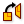Orient (Orient)
Transform  >  Euclidean  >  Orient
Orient an object. Orientation is sometimes called a 'ChangeBasis tranformation'. It allows for remapping of geometry from one axis-system to another.
Inputs
Geometry (G)Base geometry
Source (A)Initial plane
Target (B)Final plane
Outputs
Geometry (G)Reoriented geometry
Transform (X)Transformation data
component at the end of that tutorial. Now we are ready to hand the data to a CNC machine
or to add labels with dimensions and slice numbers
, which could be engraved.

## Get the results

### Version Info

• Rhino 6.31
• Grasshopper 1.0.0007

In the instructions above, the ground plane was at z = 0. Now it’s your turn to alter the algorithm in a way that we can set the altitude of the trimming plane as a variable parameter.

Hint 1

### Set a parameter for the horizontal plane

The modifications that need to be made to cut the object require little effort: First we use Construct Point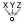Construct Point (Pt)
Vector  >  Point  >  Construct Point
Construct a point from {xyz} coordinates.
Inputs
X coordinate (X){x} coordinate
Y coordinate (Y){y} coordinate
Z coordinate (Z){z} coordinate
Outputs
Point (Pt)Point coordinate
and assign any number to input Z. The same number also needs to be connected to the Larger ThanLarger Than (Larger)
Maths  >  Operators  >  Larger Than
Larger than (or equal to)
Inputs
First Number (A)Number to test
Second Number (B)Number to test against
Outputs
Larger than (>)True if A > B
… or Equal to (>=)True if A >= B
component in the middle of step 2.

After this modification the Split BrepSplit Brep (Split)
Intersect  >  Shape  >  Split Brep
Split one brep with another.
Inputs
Brep (B)Brep to split
Cutter (C)Cutting shape
Outputs
Result (R)Brep fragments
component might be orange and warns “Split did not succeed”. This is caused by slices that are beneath the trimming plane and have no intersection. They are eliminated with the validation in step 2 and so there is nothing to worry about.

In this example, the variation of the cutting plane results in fewer slices. The old algorithm that we want to plug into does not account for that yet. Therefore, we have to remove the now redundant planes from the Plane Through Shape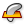Plane Through Shape (PxS)
Surface  >  Primitive  >  Plane Through Shape
Make a rectangular surface that is larger than a given shape.
Inputs
Plane (P)Surface plane
Shape (S)Shape to exceed
Inflate (I)Boundary inflation amount
Outputs
Surface (S)Resulting planar surface
component. This is a more complicated task.

Hint 2

### Remove redundant planes

When we compare the output from step 2 of this tutorial with the initial algorithm, we can see that trimming the cone sections with the elevated plane results in fewer slices. Now, we need to reduce redundant planes and the best place to start is at the output of the EntwineEntwine (Entwine)
Sets  >  Tree  >  Entwine
Flatten and combine a collection of data streams
Inputs
Branch {0;0} ({0;0})Data to entwine
Branch {0;1} ({0;1})Data to entwine
Branch {0;2} ({0;2})Data to entwine
Outputs
Result (R)Entwined result
component. It contains two branches with the planes in each direction. We use Graft Tree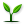Graft Tree (Graft)
Sets  >  Tree  >  Graft Tree
Graft a data tree by adding an extra branch for every item.
Inputs
Tree (T)Data tree to graft
Outputs
Tree (T)Grafted data tree
to put each plane into a branch and Tree Statistics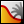Tree Statistics (TStat)
Sets  >  Tree  >  Tree Statistics
Get some statistics regarding a data tree.
Inputs
Tree (T)Data Tree to analyze
Outputs
Paths (P)All the paths of the tree
Length (L)The length of each branch in the tree
Count (C)Number of paths and branches in the tree
to get the names of the branches.

The DispatchDispatch (Dispatch)
Sets  >  List  >  Dispatch
Dispatch the items in a list into two target lists.
Inputs
List (L)List to filter
Dispatch pattern (P)Dispatch pattern
Outputs
List A (A)Dispatch target for True values
List B (B)Dispatch target for False values
component from step 2 includes all new slices, separated into branches. We can see that some branches are empty and we want to remove the corresponding planes. To identify the empty branches, we use Null Item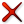Null Item (Null)
Sets  >  List  >  Null Item
Test a data item for null or invalidity
Inputs
Item (I)Item to test
Outputs
Null Flags (N)True if item is Null
Invalid Flags (X)True if item is Invalid
Description (D)A textual description of the object state
. It returns True if the branch is empty. We then use a DispatchDispatch (Dispatch)
Sets  >  List  >  Dispatch
Dispatch the items in a list into two target lists.
Inputs
List (L)List to filter
Dispatch pattern (P)Dispatch pattern
Outputs
List A (A)Dispatch target for True values
List B (B)Dispatch target for False values
component and connect the Boolean values to input P, which we also flatten. At input L we connect the names of branches from before.

At output A of Dispatch we find the names of the branches that are empty and we connect this to an input M of a Split Tree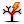Split Tree (Split)
Sets  >  Tree  >  Split Tree
Split a data tree into two parts using path masks.
Inputs
Data (D)Tree to split
Outputs
Positive (P)Positive set of data (all branches that match any of the masks)
Negative (N)Negative set of data (all branches that do not match any of the masks
component; together with the grafted section planes at input D. We can find the branches that were not included in the selection at output N. These are the planes for the non-empty sections. A Trim TreeTrim Tree (Trim)
Sets  >  Tree  >  Trim Tree
Reduce the complexity of a tree by merging the outermost branches.
Inputs
Tree (T)Data tree to flatten
Depth (D)Number of outermost branches to merge
Outputs
Tree (T)Trimmed data tree
component will put all planes in one direction into a branch.

The last part is to take a Plane Through ShapePlane Through Shape (PxS)
Surface  >  Primitive  >  Plane Through Shape
Make a rectangular surface that is larger than a given shape.
Inputs
Plane (P)Surface plane
Shape (S)Shape to exceed
Inflate (I)Boundary inflation amount
Outputs
Surface (S)Resulting planar surface
component and connect it to the rest of the algorithm, as shown in the figure.

Get the results

## Get the results

### Version Info

• Rhino 6.31
• Grasshopper 1.0.0007